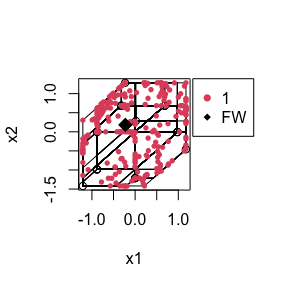# The Rtropical package

In this vignette, we will demonstrate the main capabilities of the Rtropical package. We’ll show the pipeline to analyze phylogenetic tree data with these methods.

We start by importing the Rtropical library.

library(Rtropical)
library(ape)

## Tropical SVM

We will carry out tropical SVM with the simulated tree data in the package. This data set contains 300 trees with the first 150 assumed coming from one category and the rest from the other. We firstly prepare the data by transforming it into data matrix and splitting it into training and testing set.

set.seed(101)
data(sim_trees)
treevecs = do.call("rbind", lapply(sim_trees, as.vector))
labels = as.factor(rep(c(1, 2), each = nrow(treevecs)/2))
# generate training data set
trn_ind = sample(1: nrow(treevecs), nrow(treevecs)*0.8)
x = treevecs[trn_ind, ]
y = labels[trn_ind]

# generate testing data set
newx = treevecs[-trn_ind, ]
newy = labels[-trn_ind]

Next, we run the tropical svm.

# run tropical svm
start = Sys.time()
trop_fit <- tropsvm(x, y, auto.assignment = TRUE)
end = Sys.time()
# predict for testing data
trop_pred <- predict(trop_fit, newx)
# compute classification accuracy
sum(as.vector(trop_pred) == newy)/length(newy)
#>  0.45
print(paste("The running time is: ", round(end - start, digits = 3), "s", sep = ""))
#>  "The running time is: 0.238s"

The accuracy seems to be worse because this function does not tune for a good classification method. In the cases when tropsvm fails, we recommend to use cv.tropsvm. This function automatically carries out cross-validation to improve performance. For the ease of computation, we set nassignment=100. However, users can set 500 to reach an accuracy up to 90% with running time approximately 4.5 mins.

# tropical svm with cross-validation
start = Sys.time()
cv_trop_fit <- cv.tropsvm(x, y, nassignment = 100, parallel = TRUE)
end = Sys.time()
cv_trop_pred <- predict(cv_trop_fit, newx)
# compute classification accuracy for testing data
sum(cv_trop_pred == newy)/length(newy)
#>  0.75
print(paste("The running time is: ", round(end - start, digits = 3), "min", sep = ""))
#>  "The running time is: 55.371min"

We can also run svm from e1071 as a comparison:

svm_fit <- e1071::svm(x, y)
svm_pred <- predict(svm_fit, newx)
sum(svm_pred == newy)/length(newy)
#>  0.5833333

## Tropical PCA

Now we analyze some actual phylogenetic tree data with tropical principal component analysis.

data(apicomplexa)
treevecs <- as.matrix(apicomplexa, parallel = TRUE)
pca_fit = troppca.poly(treevecs)

For second order principal component and the projection of data points, we can visualize on a 2D plane by isometric transformation.

plot(pca_fit, fw = T)# Tropical Fermat-weber Point

Fermat-weber point can be regarded as the “tropical mean” of a data set. It minimizes the sum of distance to each point in a data set, which is also the zero-th order tropical principal component analysis. Unlike tropical PCA with higher order, tropical fermat-weber point can be computed deterministically by tropFW.

tropFW(treevecs)
#> $fw #>  0.7815775 0.7815775 0.8904941 0.7815775 0.7815775 0.7815775 0.7815775 #>  0.7815775 0.7815775 0.9524738 0.9665469 0.7815775 0.7815775 0.7815775 #>  0.8353162 0.7815775 0.8837696 0.7815775 0.7815775 0.7815775 0.7815775 #>  0.7815775 0.4808759 0.6561294 0.6561294 0.6561294 0.6561294 0.0000000 #> #>$distsum
#>  434.4991